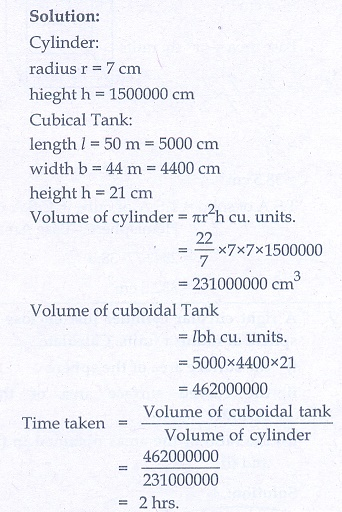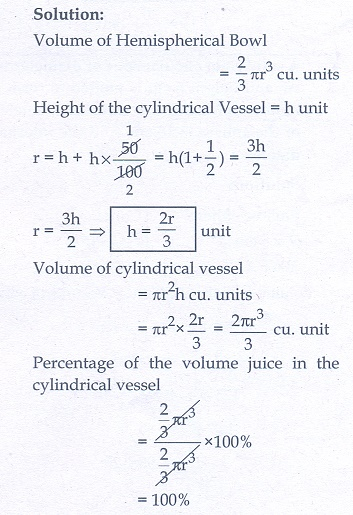Home | | Maths 10th Std | Exercise 7.4: Conversion of Solids from one shape to another with no change in Volume

# Exercise 7.4: Conversion of Solids from one shape to another with no change in Volume

Maths Book back answers and solution for Exercise questions - Mathematics : Mensuration: Conversion of Solids from one shape to another with no change in Volume: Exercise Problem Questions with Answer

Exercise 7.4

1. An aluminium sphere of radius 12 cm is melted to make a cylinder of radius 8 cm. Find the height of the cylinder.2. Water is flowing at the rate of 15 km per hour through a pipe of diameter 14 cm into a rectangular tank which is 50 m long and 44 m wide. Find the time in which the level of water in the tanks will rise by 21 cm.3. A conical flask is full of water. The flask has base radius r units and height h units, the water poured into a cylindrical flask of base radius xrunits. Find the height of water in the cylindrical flask.4. A solid right circular cone of diameter 14 cm and height 8 cm is melted to form a hollow sphere. If the external diameter of the sphere is 10 cm, find the internal diameter.5. Seenu’s house has an overhead tank in the shape of a cylinder. This is filled by pumping water from a sump (underground tank) which is in the shape of a cuboid. The sump has dimensions 2 m ×1.5 m × 1 m. The overhead tank has its radius of 60 cm and height 105 cm. Find the volume of the water left in the sump after the overhead tank has been completely filled with water from the sump which has been full, initially.6. The internal and external diameter of a hollow hemispherical shell are 6 cm and 10 cm respectively. If it is melted and recast into a solid cylinder of diameter 14 cm, then find the height of the cylinder.7. A solid sphere of radius 6 cm is melted into a hollow cylinder of uniform thickness. If the external radius of the base of the cylinder is 5 cm and its height is 32 cm, then find the thickness of the cylinder.8. A hemispherical bowl is filled to the brim with juice. The juice is poured into a cylindrical vessel whose radius is 50% more than its height. If the diameter is same for both the bowl and the cylinder then find the percentage of juice that can be transferred from the bowl into the cylindrical vessel.1. 36 cm

2. 2 hrs

3. h/3x2

4. 6 cm

5. 281200 cm3

6. 1.33 cm

7. 1 cm

8. 100%

Tags : Problem Questions with Answer, Solution | Mensuration | Mathematics , 10th Mathematics : UNIT 7 : Mensuration
Study Material, Lecturing Notes, Assignment, Reference, Wiki description explanation, brief detail
10th Mathematics : UNIT 7 : Mensuration : Exercise 7.4: Conversion of Solids from one shape to another with no change in Volume | Problem Questions with Answer, Solution | Mensuration | Mathematics